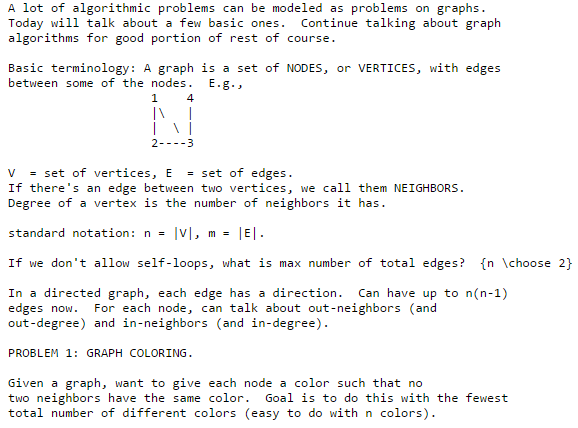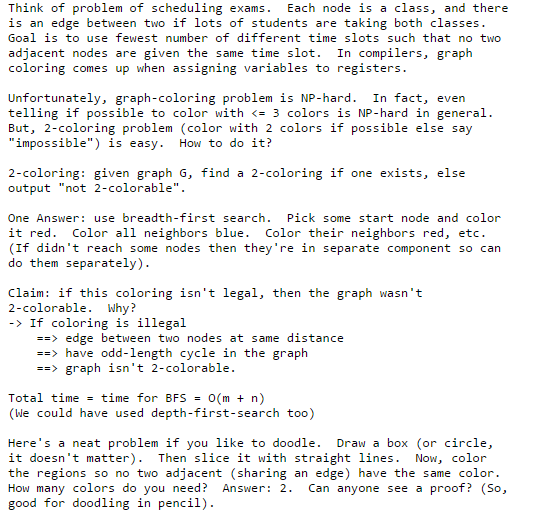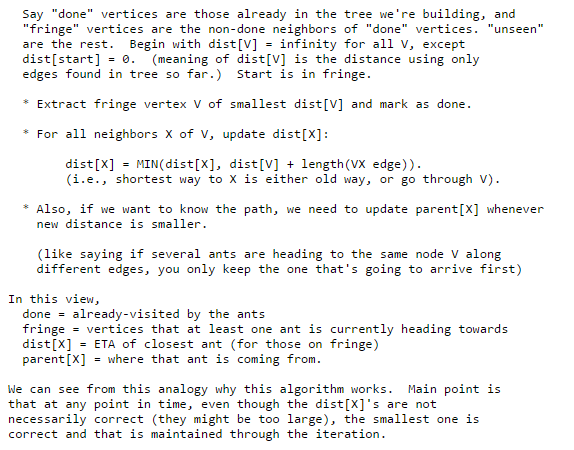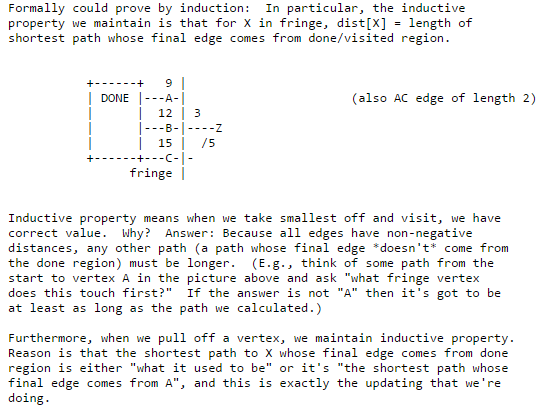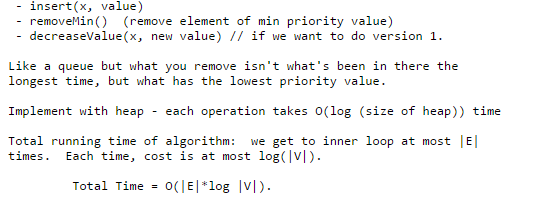# Answered! Given a graph G = (V,E) a k-coloring of the graph is a labeling of the vertices with the colors c1, c2,..,ck such that for…

Given a graph G = (V,E) a k-coloring of the graph is a labeling of the vertices with the colors c1, c2,..,ck such that for every edge (u,v) the color of the u is different from the color of v.
a. Give a linear time algorithm that finds a 2-coloring for a tree.
b. Think about coloring a tree with 2 colors so that you have the max number of nodes colored c1. Prove that the algorithm in part (a)-with a minor modification probably-can be used to solve this problem.
c. Show an example of a tree, T, that can have at most k nodes colored c1 in a 2-coloring but T can have k’>k nodes colored c1 in a 3-coloring. Try to find the smallest example of such a tree T.
d. Give an algorithm that creates a 3-coloring for a tree such that the max number of nodes are colored c1.